# Point estimator

(diff) ← Older revision | Latest revision (diff) | Newer revision → (diff)

A statistical estimator whose values are points in the set of values of the quantity to be estimated.

Suppose that in the realization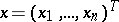of the random vector, taking values in a sample space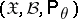,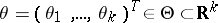, the unknown parameter(or some function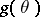) is to be estimated. Then any statistic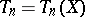producing a mapping of the set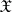into(or into the set of values of) is called a point estimator of(or of the functionto be estimated). Important characteristics of a point estimator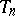are its mathematical expectationand the covariance matrix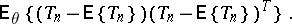The vector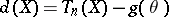is called the error vector of the point estimator. If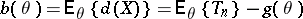is the zero vector for all, then one says thatis an unbiased estimator ofor thatis free of systematic errors; otherwise,is said to be biased, and the vectoris called the bias or systematic error of the point estimator. The quality of a point estimator can be defined by means of the risk function (cf. Risk of a statistical procedure).

How to Cite This Entry:
Point estimator. Encyclopedia of Mathematics. URL: http://encyclopediaofmath.org/index.php?title=Point_estimator&oldid=16171
This article was adapted from an original article by M.S. Nikulin (originator), which appeared in Encyclopedia of Mathematics - ISBN 1402006098. See original article